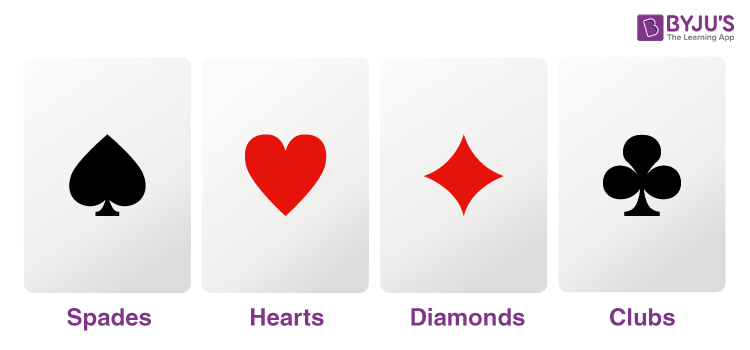Know more about the Repeaters Batch Know more about the Repeaters Batch

# How to Find Probability of Cards

The number of cards in a pack of playing cards is 52. It consists of 4 suits of 13 cards. They are spades, hearts, clubs, and diamonds. Spades and clubs are black while hearts and diamonds are red. Each suit contains an ace, king, queen, jack or knaves, 10, 9, 8, 7, 6, 5, 4, 3, and 2. King, Queen, and Jack (or Knaves) are called the face cards. In the deck of 52 playing cards, there are 12 face cards. The above explanation will help us to solve the problems of finding the probability of cards.## What is probability?

Probability shows how likely an event will happen.

The probability of an event E is defined as P(E) = Number of favourable outcomes of E/ total number of possible outcomes of E.

Or P(E) = n(E) /n(S).

## How to Find the Probability Step by Step

You can use the following steps to calculate the probability:

Step 1: Identify the number of favourable events.

Step 2: Find the total number of results that can occur.

Step 3: Divide the number of favourable events by the total number of possible outcomes.

## Formula

P(E) = n(E) /n(S).

n(E) = Number of favourable outcomes of E.

n(S) = total number of possible outcomes of E.

## Solved Examples

Example 1: A card is drawn at random from a pack of 52 playing cards. Find the probability that the card drawn is

(i) a king

(ii) neither a queen nor a jack.

Solution:

Total no. of cards = 52

So total no. of possible outcomes, n(S) = 52

(i) Let E1 denotes the event of getting a king.

No. of kings in the pack = 4

n(E1) = 4

P(getting a king) = no. of favourable outcomes / total no. of possible outcomes of E

= n(E1)/ n(S)

= 4/52

= 1/13

Hence the required probability is 1/13.

(ii) Let E2 denotes the event of getting neither a queen nor a jack.

No. of queens and jack in the pack = 8

Remaining no. of cards = 52-8 = 44

n(E2) = 44

P(neither a queen nor a jack) = no. of favourable outcomes / total no. of possible outcomes of E

= n(E2)/ n(S)

= 44/52

= 11/13

Hence the required probability is 11/13.

Example 2: A card is drawn from a well-shuffled pack of 52 cards. Find the probability of getting:

(ii) a jack

(iii) a king of red colour

(iv) a card of diamond

(v) a king or a queen

Solution:

Total no. of cards = 52

So total no. of possible outcomes, n(S) = 52

(i) Let E1 denotes the event of getting 2 of spades.

No. of ‘2’ of spades = 1

n(E1) = 1

P (getting 2 of spades) = no. of favourable outcomes/total no. of possible outcomes of E

= n(E1)/ n(S)

= 1/52

Hence, the required probability is 1/52.

(ii) Let E2 denotes the event of getting a jack.

No. of jacks in a suit = 4

n(E2) = 4

P (getting a jack) = no. of favourable outcomes/total no. of possible outcomes of E

= n(E2)/ n(S)

= 4/52

= 1/13

Hence the required probability is 1/13.

(iii) Let E3 denotes the event of getting a king of red colour.

No. of kings in red colour = 2

n(E3) = 2

P (getting king in red colour) = no. of favourable outcomes/total no. of possible outcomes of E

= n(E3)/ n(S)

= 2/52

= 1/26

Hence the required probability is 1/26.

(iv) Let E4 denotes the event of getting a card of diamond.

No. of diamond cards = 13

n(E4) = 13

P (getting a card of diamond) = no. of favourable outcomes/total no. of possible outcomes of E

= n(E4)/ n(S)

= 13/52

= 1/4

Hence the required probability is ¼.

(v) Let E5 denotes the event of getting a king or a queen.

No. of kings and queen = 4+4= 8

n(E5) = 8

P (getting a king or a queen = no. of favourable outcomes/total no. of possible outcomes of E

= n(E5)/ n(S)

= 8/52

= 2/13

Hence the required probability is 2/13.

### Give the formula to find the probability of an event.

The probability of an event E, P(E) = Number of favourable outcomes of E/total number of possible outcomes of E.

### What are the 4 types of cards?

The four types of cards are spades, hearts, clubs, and diamonds.

### What is the probability of getting a king when a card is drawn at random from a pack of 52 cards?

In a pack of 52 cards, there are 4 kings. So the required probability = 4/52 = 1/13.

### How many red cards are there in a deck of 52 cards?

There are 26 red cards.